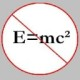You are currently browsing the tag archive for the ‘Irrationality’ tag.

The following fun problem was posed in one of the issues of the American Mathematical Monthly (if I am not wrong). I don’t remember the exact issue or the author, but here is the problem anyway.

Prove that$\sqrt[n]{2}$ is irrational for all$n > 2$ and$n \in \mathbb{N}$.

Slick solution: We could either use Euclid’s arguments or invoke the rational root theorem to prove the above statement. However, there is a slicker proof!

Assume, for the sake of contradiction, that$\sqrt[n]{2} = p/q$, where$p, q \in \mathbb{N}$ and$p \ne 0$. Then, we have$2 = (p/q)^n$ which implies$q^n + q^n = p^n$. But this contradicts Fermat’s Last Theorem! And, we are done.

### Blog Stats

• 346,162 hitsprof dr drd horia or… on My first postprof drd horia orasa… on My first postprof dr mircea orasa… on Inequality with lognotedscholar on Self-referential Paradoxes, In…prof dr mircea orasa… on Inequality with logprof dr mircea orasa… on Inequality with logprof dr mircea orasa… on 2010 in reviewkenji on Basic category theory, Iprof dr mircea orasa… on Solution to POW-10: Another ha…prof drd horia orasa… on Continued fraction for eprof drd horia orasa… on Inequality with logprof dr mircea orasa… on Solution to POW-12: A graph co…prof dr mircea orasa… on The Character of Physical…prof dr mircea orasa… on Milk and Tea puzzleprof drd horia orasa… on Inequality with log